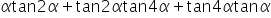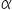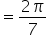Maths-
General
Easy

###Hint:

In this question, we have to find the value of tan, if. For this we will solve the function using common trigonometric identity and simplify it and later substitute the value ofin the equation.

## Book A Free Demo+91